# Rotation

A special kind of motion, for which at least one point in space remains at rest. If the rotation is in a plane, the fixed point is called the centre of the rotation; if the rotation is in space, the fixed straight line is called the axis of rotation. A rotation in a Euclidean space is called proper (a rotation of the first kind) or improper (a rotation of the second kind) depending on whether or not the orientation in space remains unchanged.

A proper rotation in a plane can be analytically expressed in Cartesian orthogonal coordinates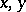by the formulas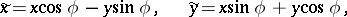whereis the rotation angle and the centre of the rotation is the coordinate origin. A proper rotation through an anglemay be represented as the product of two axial symmetries (reflections, cf. Reflection) with axes forming an angle of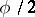with each other. An improper rotation in a plane can be analytically expressed in Cartesian orthogonal coordinatesby the formulas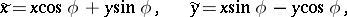whereis the rotation angle and the centre of the rotation is the coordinate origin. An improper rotation in a plane may be represented as a product of a proper rotation by an axial symmetry.

A rotation in an-dimensional Euclidean space can be analytically expressed by an orthogonal matrix in canonical form: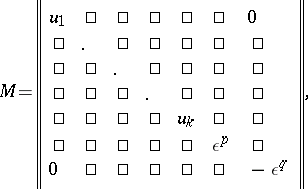where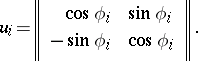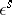is the identity matrix of order(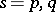). The following cases are possible:

1)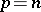— the identity transformation;

2)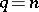— the rotation is a central symmetry;

3)— the rotation is a symmetry with respect to a-plane (a reflection in a-plane);

4)does not contain submatrices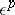and— the rotation is called a rotation around a unique fixed point;

5)contains the submatricesandbut does not contain the submatrix— the rotation is a rotation around a-plane;

6)contains the submatricesand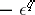but does not contain the submatrix— the rotation is called a rotational reflection in an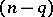-plane.

The rotations of a Euclidean space around a given point form a group with respect to multiplication of rotations. This group is isomorphic to the group of orthogonal transformations (cf. Orthogonal transformation) of the vector spaceor to the group of orthogonal matrices of orderover the field. The rotation group of the spaceis an-dimensional Lie group with an intransitive action on.